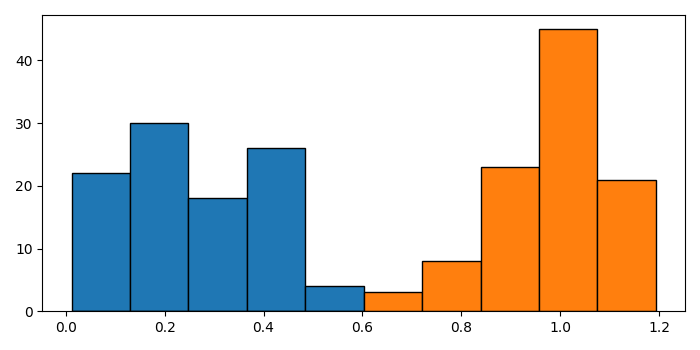# How to make two histograms have the same bin width in Matplotlib?

MatplotlibPythonData Visualization

To make two histograms having same bin width, we can compute the histogram of a set of data.

## Steps

• Create random data, a, and normal distribution, b.

• Initialize a variable, bins, for the same bin width.

• Plot a and bins using hist() method.

• Plot b and bins using hist() method.

• To display the figure, use show() method.

## Example

import numpy as np
from matplotlib import pyplot as plt
plt.rcParams["figure.figsize"] = [7.00, 3.50]
plt.rcParams["figure.autolayout"] = True
a = np.random.random(100) * 0.5
b = 1 - np.random.normal(size=100) * 0.1
bins = 10
bins = np.histogram(np.hstack((a, b)), bins=bins)
plt.hist(a, bins, edgecolor='black')
plt.hist(b, bins, edgecolor='black')
plt.show()

## Output